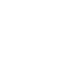# SAT & ACT Question of the Day: Nov. 27, 2013

If you are reading this in an email you received from me, do not click the link to sat.collegeboard.org below. Use the link to my website that is farther down on the email.

If you are seeing this in my blog, do the SAT Question of the Day by clicking on this link:

http://sat.collegeboard.org/practice/sat-question-of-the-day?questionId=20131127&oq=1 (This link takes you to today’s question. If you use my archive, you will see the question related to my SAT explanation for that date.)

This is a great question.  It can teach us a lot about the SAT and ACT tests.

The answer is C.  Take a look at the test writers’ explanation and you’ll see one way, albeit a long way, to do the question.  Nothing wrong with their way; in fact, once you realize it is a 30:60:90 right triangle, it is pretty fast.  Many students won’t notice that.

There’s another way to do it that may be easier for you.  Anytime there is a triangle inside a circle and one side is the diameter and the third vertex of the triangle lies on the circumference as it does for Triangle ABC, then the third angle (B) has to be a right angle.  (This is “Thales’ Theorem but poor old Thales wasn’t as famous as Pythagoras who sometimes gets credit for Thales’ clever calculation!)

Anyway, given that ABO is equilateral and the sides are 6, AB is 6 and AC is 12.  Knowing that angle ABC is a right angle we can use the Pythagorean Theorem to solve the puzzle:  c2 – a2 = b2.   122 – 62 = b2.  144 – 36 = 108.
Finally, √108 = 6√3.

Trick: If you can’t quickly factor √108 to convert it to 6√3, there’s a couple of things you can do.  One easy thing to do is use your calculator to determine the square root of 108, 10.4, or you know that since the square root of 100 is 10 and the square root of 121 is 11, the square root of 108 has to be slightly closer to 10 than it is 11 (less than 1.5).  Now check the answers starting with C because it is in the middle of a bunch of numbers that are in ascending order.  (The test writers violate that normal pattern today by putting all the radical 3 answers together.)  Use your calculator to multiply 6 times the square root of 3 is about 10.4.  Boom–all done.  I cheated by doing something easier!  Growing up in the “dark ages” before calculators, I had to memorize the square roots of 1 through 9.  I knew that √3 is about 1.7 (not quite 2 because the square root of 4 is 2) and 6 times 1.7 is going to be a little more than 10!  No fuss, no muss and much faster than putting numbers into a calculator.

You could also avoid doing any math for this question or at least check your work by using the fact that on the SAT (not the ACT) the diagrams are drawn to scale unless they tell you otherwise.  AC =12 and BC is slightly shorter than AC.  Answers C and D are visually close enough to guess between and the other answers are way off, eliminate them.  Now let’s figure out between C and D.  9 is from point A and half way from O to C  (1.5 radii!).  You can see BC is longer than that length; so, C has to be correct.  That was sure easy!

What are the things we can learn from this question that will help on test day?

• First, always remember to use a simple fact that all radii are equal and the diameter is twice the radius.  Any two radii that create a triangle with the third side being a chord that connects the two points where the radii intersect the circumference form an isosceles triangle!  Triangle ABO is a special case of an isosceles because the angle at O is 60 degrees.
• Second, had they told you angle AOB is 60 degrees, you could have figured out chord AB is equal to the radius and the triangle has to be an equilateral.  (Just memorize that fact.)
• Third, while he isn’t as popular as Pythagoras, Thales’ Theorem is also very valuable to know.
• Fourth, the SAT test writers love triangles and especially right triangles.  They love to hide them on the test.
• Fifth, there’s almost always more than one way to do the math questions.  There were at least three for this one.  Which one worked best for you?

Let’s see what the ACT folks have for us this morning.

I’m not going to spend much time explaining this question because we just saw it a couple of weeks ago.  I figure if I can remember the right answer without reading the passage, then it’s not worth wasting your time or mine.

The answer is D.  Roman Numeral II disagrees with the story–eliminate it.  I and III certainly follow the PICK rules (see my free website or check out Video #3 for a full explanation).

If you are interested in the online videos, you can go to my homepage and click on the online video course button to get a special registration of \$1 for three days.  Give it a try.

Tomorrow should be a great day: Family, Friends, Food, and Football–enjoy your time off school.

The SAT & ACT Wizard